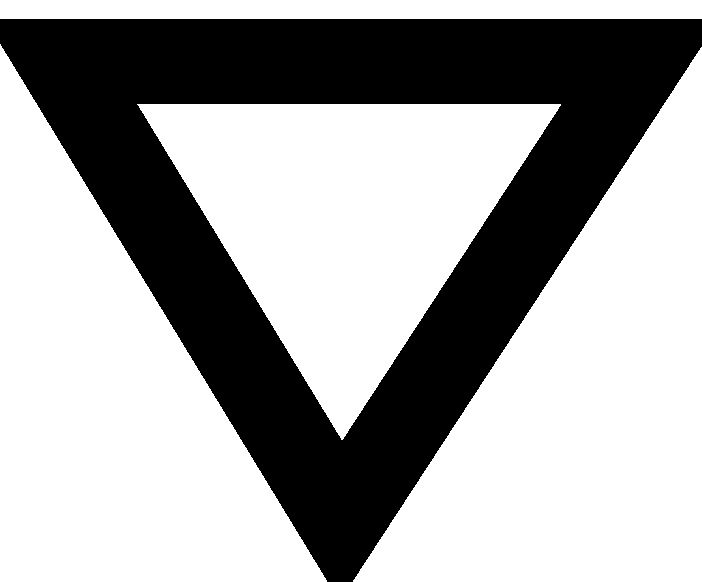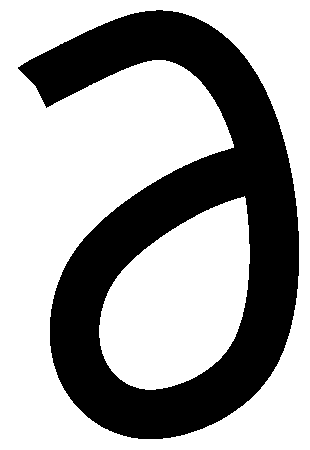Answers to the review problems for the first exam, 251:05-10 in spring 2006

Problems with letters whose backgrounds are this color have
answers here. Those with backgrounds in this color do not.

Have you done a problem?

 A B C D E F(part a) only) G & H I J K L M N O & P(parts a,b, and c) Q RIn the diary S T UIn the diary V W & X

Answers contributed by section
5678910
612123

A
Contributed by Matthew Starr of Section 5:
Sketch two level curves of f(x,y) = (x-y)/(x+y). Explain why lim
(x,y)->(0,0) (x-y)/(x+y) does or does not exist.

Original function: f(x,y) = (x-y)/(x+y)
Set y =x, curve: f(x,x) = (0)/(2x)    =0 no matter what x approaches
Set y  =2x, curve: f(x,2x) = (-1x)/(3x) = (-1/3) no matter what x
approaches
Because these limits are not the same (including when they approach
(0,0) ) the limit does not exist.
Comment The management offers this about the graphical part of the problem:
Sketch two level curves of f(x,y) = (x-y)/(x+y).
For example, we could consider (x-y)/(x+y)=0. Then we get x-y=0 so that y=x "is" the level curve. (Actually, more precisely, I guess (0,0) is not on the level curve, since "0/0" is not defined. How about another level curve? Let's try (x-y)/(x+y)=1. Then x-y=x+y so the 2y=0 and y=0. This is the x-axis (except, again, for (0,0)). Just look at these two level curves should advise you that something weird is happening at (0,0): there are points really close to (0,0) where f's values are both 0 and 1, so I bet the limit doesn't exist.

B
Contributed by Erin Curcio of Section 7:

B. Let f(x,y)=xy2 + 2x3

a)If one stands on the graph of f at (1,2,6), what is the slope of the
graph at that point in its steepest direction? Please see comment below.

I don't know exactly how you would find this, but I think you would
need to begin by finding the tangent plane because that would be
similar to the slope. To do this find the partial derivatives.

fx(x,y)=y2 +6x2      fy(x,y)=2xy

The tangent plane at (1,2,6) would be:

z-6=10(x-1) + 4(y-2)

Comment The management declares that this problem was copied from another instructor's exam. I think that the instructor wanted "the slope of the graph" to mean the directional derivative in that direction. Sigh. In this problem,f=<y2+6x2,2xy>. At (1,2,6) this would be <10,4> so the largest value of Duf=f·u is sqrt(102+42=sqrt(116). The u involved would be <10/sqrt(116),4/sqrt(116)>
b)Find Duf(1,2) if u is the unit vector in the direction from (1,2) to (2,4). The length of the vector is found to be (1,2) by subtracting the first point from the second. To find the unit vector, divide the length by the magnitude. |u|=(1·2 + 2·2).5 = (5).5 so, the unit vector is <1/(sqrt 5), 2/(sqrt 5)> Du(x,y)=fx(x,y)a + fy(x,y)b = (y2+6x2)(1/sqrt 5)+(2xy)(2/sqrt5) Du(1,2)=(10/sqrt5)+(8/sqrt5)=18/(sqrt5)

C
Contributed by Matthew Starr of Section 5:

Find an equation of the plan containing the points (1,2,0) and (0,2,1)
and parallel to the line x=1+t, y=-1+t, z=2t

(these are x,y,z coordinates of a point on the plane)
|          |          |
v          v          v
The equation of a plane is __(x-__) + __(y-__) + __(z-__) = 0
^         ^           ^
|         |           |
(Vector components of line
perpendicular to plane)

Using the parametric equation of the parallel line, we know that a
vector in its direction is &;lt;1,1,2>. We should use it because
it represents the parallel aspect.
The equation of a plane requires a perpendicular line, Find another
parallel vector and then cross product the <1,1,2> with it to produce
a perpendicular vector.

(0-1)i+(2-2)j+(1-0)k
This is the vector created when we use the two points given to us that
lie on the plane.
Now cross product the two vectors
<1, 1, 2> x <-1, 0, 1>=<1,-3,1>
Plug these values into the plane equation:
1(x-1) + (-3)(y-2) + 1(z-0) = 0

D
Contributed by Matthew Starr of Section 5:

Z= f(x,y), x = 3uv, y = u-v^2, express Dz/Dv in terms of Dz/Dx, Dz/Dy,
u, and v.

Z=(f(x,y))
(Dz/Dv) = (Dz/Dx)(Dx/Dv) + (Dz/Dy)(Dy/Dv)
(Dz/Dv) = (Dz/Dx)(3u) + (Dz/Dy)(-2v)

E
Contributed by Matthew Starr of Section 5:

x5+y5+z3+xyz=0 find an equation of the tanget line at
(1,1,-1) and the value of Dz/Dx at (1,1,-1)

Formula:   Fx(Xo,Yo,Zo)(x-Xo)+(Fy(Xo,Yo,Zo)(y-Yo)+Fz(Xo,Yo,Zo)(z-Zo) =0

Here (1,1,-1) is (xo,yo,zo).
Fx: ((5(x4)+(yz)) at (1,1,-1) is 4
Fy: (5(y4) at (1,1,-1) is 5
Fz: (3(z2)+(xy)) at (1,1,-1) is 4

Contributed by Adam Skrzypczak of Section 10:

Just/z the whole equation.

Remember that y is constant and z is supposed to be a function of x (use the chain rule!).

5(x4)+3z2[z/x]+yz+xy[z/x]=0

So (3z2+xy)[z/x]=-5x4-yz

and [z/x]=(-5x4-yz)/(3z2+xy)

At the point (1,1,-1) this is (-5+1)/(3+1)=-1.

F
Contributed by David Yakubov of Section 10:

f(t)=sin(2t)i+tj+t^3k
1) Set up a sytem of equations
sin(2t)=0
4/t=0
t^3=0,     this implies that t=0
do the same for (0,pi,pi^3) and we will get t=pi

2) Take the first derivative of the function, r'(t)=2cos(2t)i+j+3t^2k
lr'(t)l=sqrt(4cos^2(2t)+9t^4+1)

3) Set up an integral from 0 to pi of sqrt(4cos^2(2t)+9t^4+1) dt

G & H
Contributed by Annemarie Manfredi of Section 10:

a) V + 2W = <1,3,-2> + 2<-1,2,1>
<1,3,-2> + <-2,4,2>
<1 + -2, 3 + 4, -2 + 2>
V + 2W = <-1, 7, 0>
vector/magnitude creates a unit vector so:
|V+2W| = sqrt(-12 + 72 + 02)
|V+2W| = sqrt(1 + 49)
|V+2W| = sqrt(50)

unit vector in the same direction as V + 2W: <-1,7,0>/(5sqrt2)

b) magnitude of V1||W = V·W/|W|
V·W = -1 + 6 -2=3
|W| = sqrt(1+4+1)
|W| = sqrt(6)
V1 = 3/(sqrt6) multiplied by a unit vector in the direction of W,
and that unit vector is W/|W|=<-1,2,1>/sqrt(6) so
V1=<-1,2,1>/sqrt(6) times 3/sqrt(6)
V1=<-1,2,1>(1/2)=<-1/2,1,1/2>

Since V = V1 + V2
V2 = V - V1
V2 = <1,3,-2> - <-1/2,1,1/2> = <3/2,2,-5/2>

Comment The management notes that the answer can be checked independently by computing V2·W=<3/2,2,-5/2>·<-1,2,1>=(-3/2)+4+(-5/2)=0, so they are perpendicular. If you have the time this is a good idea.

K
Contributed by Umesh Kishnani of Section 8:

Answer to part a of problem K:

z + xz/x + cos(y^2 + z^2) (2z)z/x = 0z/x [x + 2zcos(y^2 + z^2)] = -zz/x = -z / [x + 2zcos(y^2 + z^2)]

L
Contributed by Tina H. Lee of Section 6:

r(t)=(cos(2t)+2t*sin(2t))i+(sin(2t)-2t*cos(2t))j

r´(t)=<-2sin(2t)+2sin(2t)+4t*cos(2t),2cos(2t)-(2cos(2t)+4t*sin(2t))>=<4t*cos(2t),4t*sin(2t)>

|r´(t)|2=(16*t2)*((cos(2t))2+(sin(2t))2)=16*t2
so |r´(t)|=4t

T(t)=<4t*cos(2t),4t*sin(2t)>/(4t)=<cos(2t),sin(2t)>

T´(t)=<-2sin(2t),2cos(2t)>=<

|T´(t)|2=22(sin(2t))2+22(cos(2t))2=22
so |T´(t)|=2

N(t)=(1/2)<-2sin(2t),2cos(2t)>=<-sin(2t),cos(2t)>

k=|T´(t)|/|r´(t)|=2/(4t)=1/(2t)

J
Contributed by Matthew Starr of Section 5:

Find an equation of the tangent plane to the surface z = (x3)(y) at
(1,2,2)

Equation z-Zo=fx(Xo,Yo)(x-Xo)+fy(Xo,Yo)(y-Yo)

Now fx=3x2y at (1,2,2) this is 6
fy=x3 at (1,2,2) this is 1

z-2 = 6(x-1) + 1(y-2)

M
Contributed by Chris Martin of Section 9:

A particle's acceleration is a(t) = <cos(t), sin(t), 2>.
Suppose that r(0) = <1,0,1>
v(0) = <0,2,0>
What is r(t) = ?

v(t) = <sin(t)+const, -cos(t)+const, 2t+const>
sin(0)+const=0        -cos(0)+const=2        2(0)+const=0

v(t) = <sin(t), -cos(t)+3, 2t>

r(t) = < -cos(t)+const, -sin(t)+3t+const, (t2)+const>
-cos(0)+const=1      -sin(0)+3t+const=0     (02)+const=1

r(t) = < -cos(t)+2, -sin(t)+3t, (t2)+1>

O & P
Contributed by Sonam Patel of Section 5:

O and P part a and b

a) r(t)=costi+sintj+t2k

To find unit tangent vector to C when t=Pi, first take the derivative
of r(t) and then
take the magnitude of the derivative of r(t)

r'(t)=-sinti+costj+2tk
magnitude of r'(t)= square root of ((sint)2+(cost)2+(2t)2) = square
root of (1+4t2)

now,
r'(t)/magnitude of r'(t) will give you the unit tangent vector, so

r'(t)/magnitude of r'(t)= <-sint, cost, 2t>*1/(square root of
(1+4t2))

r'(Pi)/magnitude of r'(Pi)=<0, -1/(square root of (1+4Pi2)),
2Pi/(square root of
(1+4Pi2))> = unit tangent vector when t=Pi

b)Write an integral for the length of the part of C between t = 0 and
t = 2Pi

L= the integral from a to b of the magnitude of r'(t) dt
L= the integral from 0 to 2Pi of [square root of (1+4t2)]dt

The following was contributed by Contributed by Estee Orani of Section 7:
c)
both r'(t) and |r'(t)| are calculated above .  r''(t)= -cos t i -sin t
j +2k r'(t)×r''(t)= (2 cos t - -2t sin t) i+ (-2t cos t - -2 sin t) j
+ k=(2 cos t +2t sin t) i+ (-2t cos t+ 2 sin t) j + 1k
|r'(t)×r''(t)|= sqrt(5+t2) (The
management can't copy Ms. Orani's equisitely beautiful
computations because they are in some sort of horrible Microsoft
format. Yes, this is editorial comment. The computations use
cos2+sin2)=1 a bunch of times.)  Then the
desired formula for curvature follows by dividing by
|r'(t)|3.

S
Contributed by Thomas Schirripa of Section 9:

(a) Find parametric equations for the line through the points (3,2,7) and (-1, 1,2).
A line in R3 is defined by a point and a vector in the direction
of the line. Note that the two given points may be rewritten as position
vectors, namely: <3,2,7> and <-1,1,2>. The vector in the
direction of the line directed through these two points is just the
difference between the vectors (and vector subtraction is done
componentwise):
<3,2,7> - <-1,1,2> = <3-(-1), 2-1, 7-2> = <4,1,5>
Now pick either of the points (I used the first) and plug them into
the  "formula" for parametric equations.
x = 3 + 4t
y = 2 + 1t
z = 7 + 5t
(In case you forgot, the "formula" is
<x,y,z>=<Xo,Yo,Zo>+t<direction>, where the subscript "o" corresponds to
an initial value from a point that is on the line).

(b) At what point does this line intersect the plane x + y + z = 22?
Simply substitute the parametric equations obtained in (a) into the
equation of the plane (and solve for t):
(3 + 4t) + (2 + 1t) + (7 + 5t) = 22
12 + 10t = 22
10t = 10
t = 1
THE PROBLEM IS NOT FINISHED! The question asks for the POINT, and right
now we only obtained the t value that describes this point. By
substituting 1 for t in the parametric equations found in (a), we get (7, 3, 12).

 ... There have been many studies of elite performers -- concert violinists, chess grand masters, professional ice-skaters, mathematicians, and so forth -- and the biggest difference researchers find between them and lesser performers is the amount of deliberate practice they've accumulated. Indeed, the most important talent may be the talent for practice itself. K. Anders Ericsson, a cognitive psychologist and an expert on performance, notes that the most important role that innate factors play may be in a person's willingness to engage in sustained training. He has found, for example, that top performers dislike practicing just as much as others do. (That's why, for example, athletes and musicians usually quit practicing when they retire.) But, more than others, they have the will to keep at it anyway.

 (From "The Learning Curve" by Atul Gawande, an article on the training of surgeons appearing on pages 52-61 of The New Yorker, January 28, 2002.) The article is also included in Complications, A Surgeon's Notes on an Imperfect Science, an interesting collection of Gawande's essays.

Maintained by greenfie@math.rutgers.edu and last modified 9/5/2005.